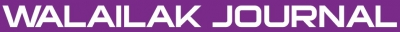### A Fractional Model of Bloch Equation in Nuclear Magnetic Resonance and its Analytic Approximate Solution

Sunil KUMAR, Naeem FARAZ, Khosro SAYEVAND

#### Abstract

The purpose of this paper is to employ an analytical approach to the time fractional Bloch Nuclear Magnetic Resonance (NMR) flow equations. A comparative study of the numerical solutions and the well-known analytical solutions are discussed. Absolute error has been calculated to show the accuracy of the applied method. The numerical solutions show that only a few iterations are needed to obtain accurate approximate solutions. The fractional derivatives are described in the Caputo sense. Numerical results are presented graphically.

doi:10.14456/WJST.2014.44

#### Keywords

Bloch equation, Caputo derivative, analytical solution, homotopy perturbation method

PDF

#### References

F Bloch. Nuclear induction. Phys. Rev. 1946; 70, 460-74.

HC Torrey. Bloch equations with diffusion terms. Phys. Rev. 1956; 104, 563-5.

J Jeener. Dynamical effects of the dipolar field in homogeneities in high-resolution NMR: spectral clustering and instabilities. Phys. Rev. Lett. 1999; 82, 1772-5.

DE Rourke, L Khodarinova and AA Karabanov. Two-level systems with relaxation. Phys. Rev. Lett. 2004; 92, 1-4.

I Petras. Modelling and numerical analysis of fractional order Bloch equations. Comp. Math. Appl. 2011; 61, 341-56.

S Bhalekar, GV Daftardar, D Baleanu and R Magin. Fractional Bloch equation with delay. Comput. Math. Appl. 2011; 61, 1355-65.

AD Bain, CK An and Z Nie. Exact solution to the Bloch equations and application to the Hahn echo. J. Magn. Res. 2010; 206, 227-40.

OB Awojoyogber. Analytical solution of the time dependent Bloch NMR, flow equations: a translational mechanical analysis. Phys. A Stat. Mech. Appl. 2004; 339, 437-60.

OB Awojoyogber and K Boubaker. A solution to Bloch NMR flow equations for the Analysis of hemodynamics functions of blood flow system using m-Boubaker polynomials. Curr. Appl. Phys. 2009; 2, 278-83.

K Murase and N Tanki. Numerical solutions to the time dependent Bloch equations revisited. Magn. Res. Imag. 2011; 29, 126-31.

S Balac and L Chupin. Fast approximate solution of Bloch equation for simulation of RF artifacts in Magnetic Resonance Imaging. Math. Comput. Model. 2008; 48, 1901-13.

JC Leyte. Some solutions of the Bloch equations. Chem. Phys. Lett. 1990; 165, 213-20.

DI Hoult. The solution of the Bloch equation in the presences of a varying B1 field - An approach to selective pulse analysis. J. Magn. Res. 1979; 35, 69-86.

H Yan, B Chen and JC Gore. Approximate solutions of the Bloch equations for selective excitation. J. Magn. Res. 1987; 75, 83-95.

J Schotland and JS Leigh. Exact solution of the Bloch equations with n-site chemical exchange. J. Magn. Res. 1983; 51, 48-55.

EA Sivers. Approximate solution to the Bloch equation with symmetric RF pulses and flip angles less than TT/2. J. Magn. Res. 1986; 69, 28-40.

Z Xu and AK Chan. A Near-Resonance Solution to the Bloch equations and its application to RF pulse design. J. Mag. Res. 1999; 138, 225-31.

KB Oldham and J Spanier. The Fractional Calculus. Academic Press, New York, 1974.

KS Miller and B Ross. An Introduction to the Fractional Calculus and Fractional Differential Equations. John Willey and Sons, New York, 2003.

I Podlubay. Fractional Differential Equations Calculus. Academic, Press, New York.

AA Kilbas, HM Srivastava and JJ Trujillo. Theory and Application of Fractional Differential Equations. Elsevier, Amsterdam, 2006.

JH He. Homotopy perturbation technique. Comput. Meth. Appl. Mech. Eng. 1999; 178, 257-62.

JH He. A coupling of method of homotopy technique and perturbation technique for nonlinear problems. Int. J. Nonlinear Mech. 2000; 35, 37-43.

JH He. Comparison of homotopy perturbation method and homotopy analysis method. Appl. Math. Comput. 2004; 156, 527-39.

JH He. Homotopy perturbation method for solving boundary value problems. Phys. Lett. A 2006; 350, 87-8.

A Yildirim and H Kocak. Homotopy perturbation method for solving the space time fractional advection dispersion equation. Adv. Water. Resour. 2009; 32, 1711-6.

A Yildirim and Y Gulkanat. Analytical approach to fractional Zakharov-kuznetsov equations by He’s homotopy perturbation method. Commun. Theor. Phys. 2010; 53, 1005-10.

NA Khan, NU Khan, M Ayaz and A Mahmood. Analytical methods for solving the time fractional Swift Hohenberg (S-H) equation. Comput. Math. Appl. 2011; 61, 2182-5.

S Kumar and OP Singh. Numerical inversion of Abel integral equation using homotopy perturbation method. Z. Naturforsch 2010; 65a, 677-82.

Q Wang. Homotopy perturbation method for Kdv equation. Appl. Math. Comput. 2007; 90, 1795-802.

KA Gepreel. The homotopy perturbation method applied to the nonlinear fractional Kolmogorov- Petrovskii-Piskunov equations. Appl. Math. Lett. 2011; 24, 1428-34.

F Mainardi. On the Initial Value Problem for the Fractional Diffusion-Wave Equation. In: S Rionero and T Ruggeeri (eds.). Waves and Stability in Continuous Media, World Scientific, Singapore, 1994, p. 246-51.

### Refbacks

• There are currently no refbacks.Online ISSN: 2228-835X

http://wjst.wu.ac.th

Last updated: 20 June 2019